Select Page
May 6, 2021Author: José M. Saavedra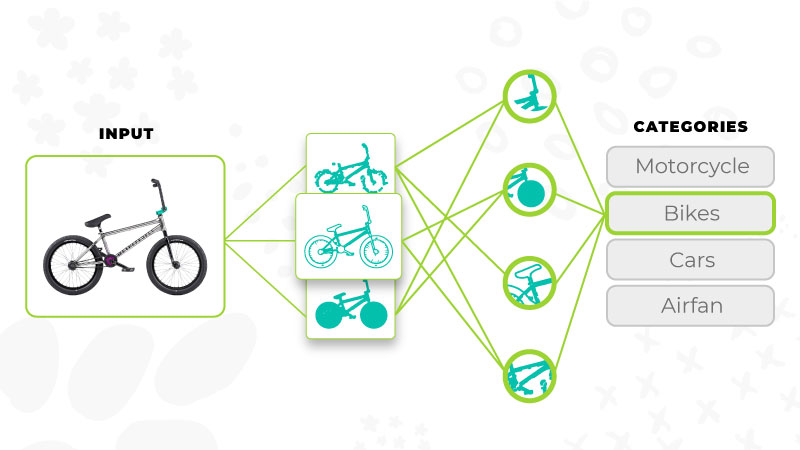If you are trying to create a model for classifying images and have a set of examples for training, you should try a convolutional neural network. Knowing that training a convnet could be somehow struggling, I wrote this blog to help you in your first touch with this technology. Here, I will teach you how to train a customized convolutional neural network for image classification.

## The repository

First of all, you will need to download our convolutional neural network repository, which is based on Tensorflow2.3 with Keras. In this repository, you will find implementations of well-known architectures like AlexNet and ResNet. Moreover, to facilitate the data manipulation, we will store it as tfrecords (a nice format of Tensorflow) that are then read by a Dataset object. This allows the model to accelerate the reading process during training.

## The example dataset

To make this example easy, we will use the well-known MNIST dataset during this blog. Please download the training and testing datasets. It consists of 60000 images for training and 10000 images for testing. Figure 1 shows a sample of the images we can find in the MNIST dataset.

If you want to try with a smaller dataset, you can use MNIST-5000, which is a sample of MNIST with 5000 images for training.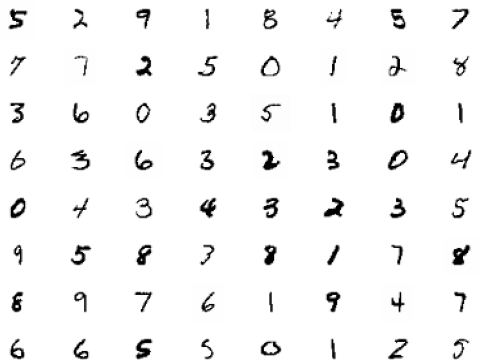Figure 1. A sample of images from the MNIST dataset.

## Prepare the dataset for training

We will require two text files, one listing the images that will be used for training and the other listing the images for testing (or validation). These files should be named as train.txt and test.txt, respectively. These should be in a two-column format, where the first column describes the absolute filename for each image, and the second is for the corresponding class (0-indexed). The separator between columns is the tab character. Finally, to organize the files, we will call DATA_DIR the folder where the described text files are located.

## Prepare a configuration file

Training a convnet requires defining a set of hyperparameters. A good manner of tuning these parameters is through a configuration file, where we define the parameters required for the training and testing stages. In this toolkit, we encourage users to create a configuration file where they can set parameters like:
• NUM_EPOCHS: The number of epochs the training will run.
• NUM_CLASSES: The number of classes in the classification task.
• BATCH_SIZE: The size of each batch.
• VALIDATION_STEPS: The number of iterations required to cover the validation dataset. It can be calculated as the size of the validation dataset divided by the batch size.
• LEARNING_RATE: The learning rate value or the initial learning rate in case of using decay schedule.
• SNAPSHOT_DIR: A path where weights are stored during training.
• DATA_DIR: The path where train.txt and test.txt are located.
• CHANNELS: The number of channels for the input images.
• IMAGE_TYPE: It is a name defining the
preprocessing operations to be applied to the input images.
• IMAGE_WIDTH: The target image width.
• IMAGE_HEIGHT: The target image height.
• SHUFFLE_SIZE: It is a number representing the size of memory reserved for shuffling the data for each epoch.
• CKPFILE: It is an absolute path identifying a checkpoint from which weights are loaded for initialization. It is optional

These parameters define one experiment but we can include different sets of parameters for various experiments. To identify each set of parameters we use a section name. An example of a configuration file (mnist_full.config) that we will use for the MNIST dataset is shown below:

[MNIST]

• NUM_EPOCHS = 10
• NUM_CLASSES = 10
• BATCH_SIZE = 128
• VALIDATION_STEPS = 78
• LEARNING_RATE: The learning rate value or the initial learning rate in case of using decay schedule.
• SNAPSHOT_DIR = <PATH>
• DATA_DIR = <PATH>
• CHANNELS =.1
• IMAGE_TYPE = MNIST
• IMAGE_WIDTH = 31
• IMAGE_HEIGHT =  31
• SHUFFLE_SIZE = 10000

## Create tfrecords

An efficient way to store the data is through tfrecords. This allows the data to efficiently feed the underlying neural network. In our example we create the tfrecords file using the following command:

#### \$python datasets/create_tfrecords.py -type all -config configs/mnist_full.config -name MNIST

With the outputs produced by the previous step, we are ready to train the model. We define a simple architecture composed of two convolutional layers at the beginning, and two fully connected layers at the end. The last layer is the classification layers with as many neurons as classes the task has. The implementation of this architecture can be found here.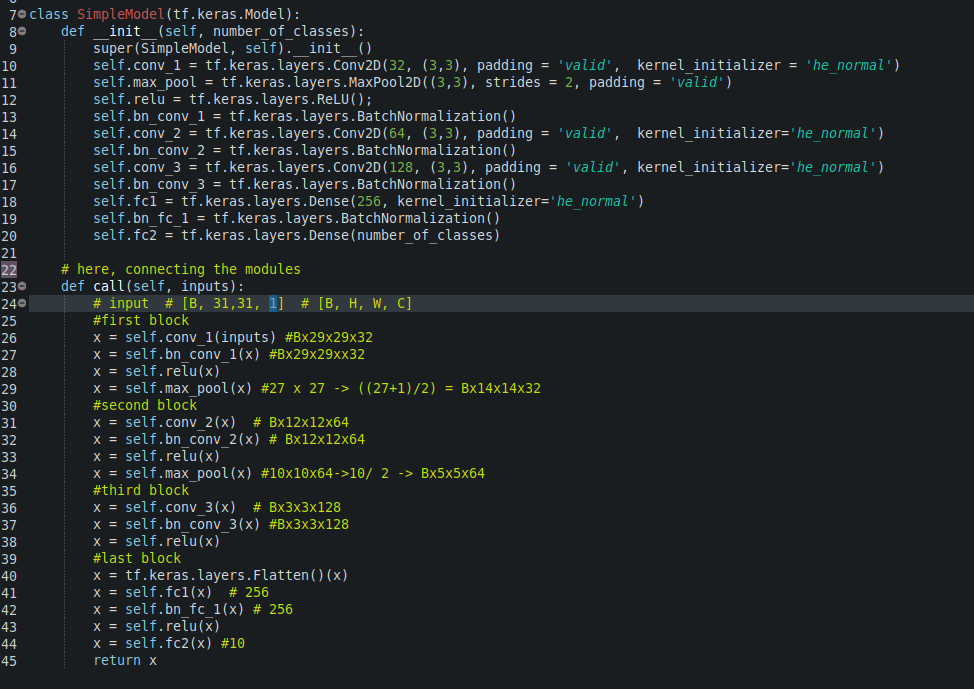Figure 2.  An implementation of a simple convnet for MNIST.

Now we are ready to train our model. You can do it running the following command:

#### \$python train_simple.py -mode train  -config configs/mnist_full.config -name MNIST

At the beginning of the execution, you will see a report of the involved parameters. Then, you will see the performance of the training for each iteration showing the loss and accuracy of the model. At the end of each epoch, the same metrics are reported for the testing (validation) dataset.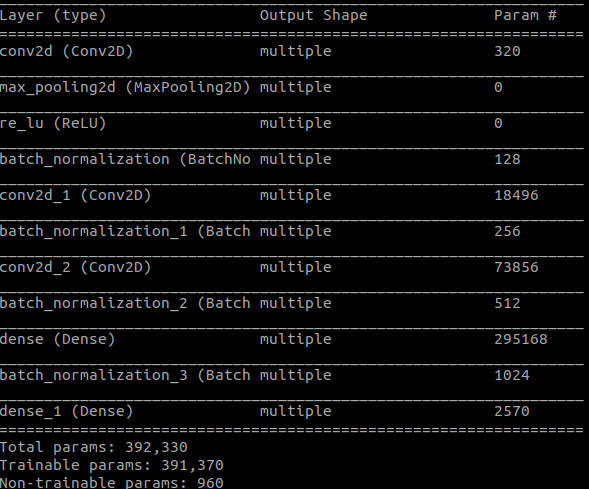Figure 3.  Parameters required for the network.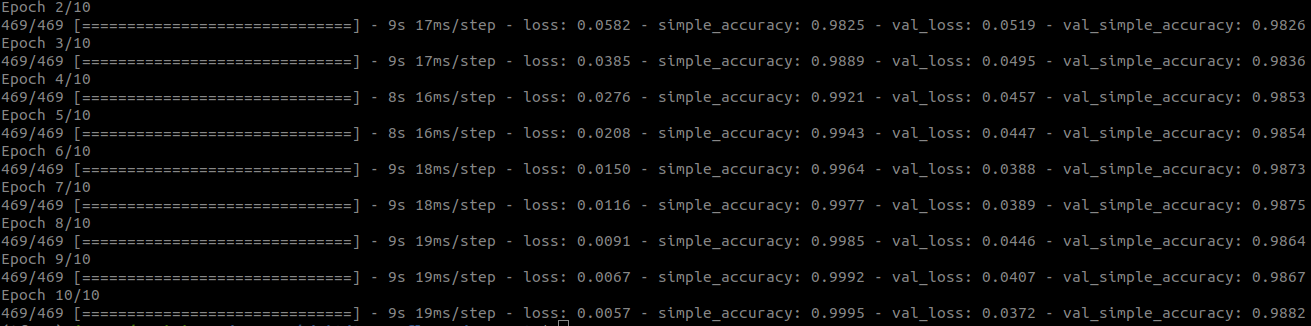Figure 4.  Output produced during training.

The training process will yield checkpoints after each epoch. In this particular case, you will see 10 files named from 001.h5 to 010.h5

You can also test your model. To this end, you need to set the CKPFILE parameter in the configuration file with the checkpoint you want to try (e.g. 010.h5), and then run the command below, which is very similar to the previous one changing the parameter mode for testing.

#### \$python train_simple.py -mode test  -config configs/mnist_full.config -name MNIST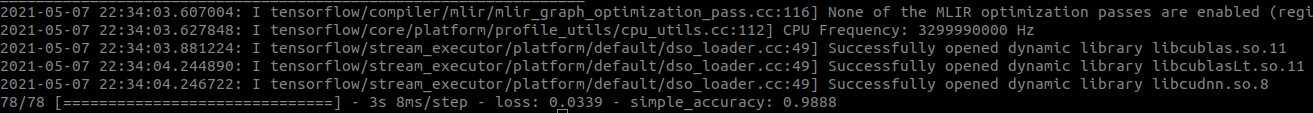Figure 5.  Output produced by testing.

## Predict with your trained model

#### \$python train_simple.py -mode predict  -config configs/mnist_full.config -name MNIST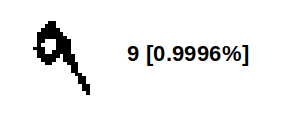Figure 6.  Example of a prediction of the MNIST model.

# Understanding the code

The code in train_simple.py is composed of the following sections:

In this section, the tfrecord file is fed into a dataset object, which allows us to organize the data into batches, apply data augmentation using the function map, and shuffle the data after each epoch.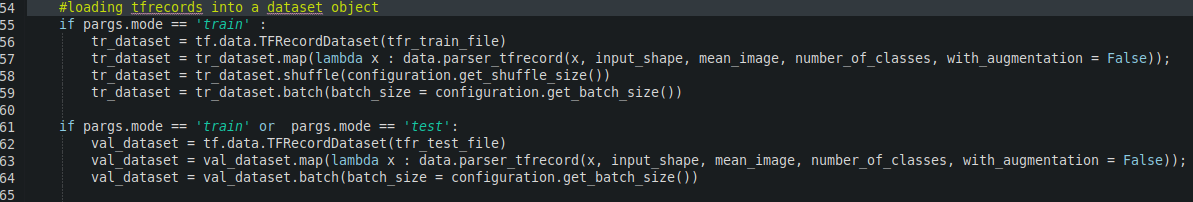## Define callbacks for saving checkpoints

Here, we configure a ModelCheckpoint object to save checkpoints after a certain number of iterations during training (e.g., after each epoch). Check the ModelCheckpoint object for more details.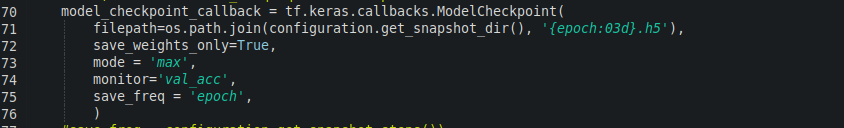## Create an instance of your network

Here, we create an instance of the network you will use. In this example, we create an instance of SimpleModel. The last part of this section builds the model defining the input size.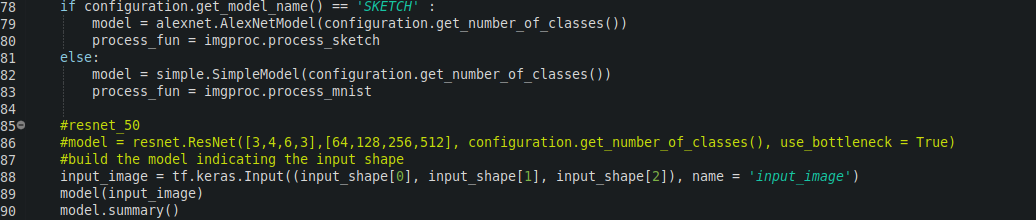Optionally, you can load pre-trained weight using the function load_weights of the model.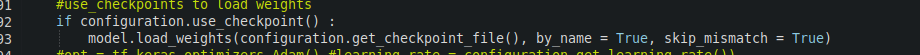## Defining the optimizer

In this section, you need to define the optimizer for your model. In this example, we are using SGD with Nesterov momentum.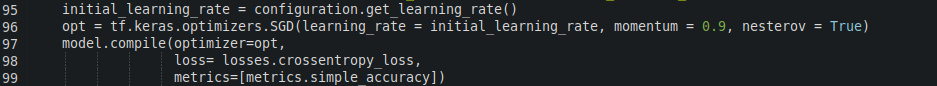## Train, test or predict

The last section of the code is devoted to training the model. Then, if you already have a trained model, you can test your model or perform predictions with some input images.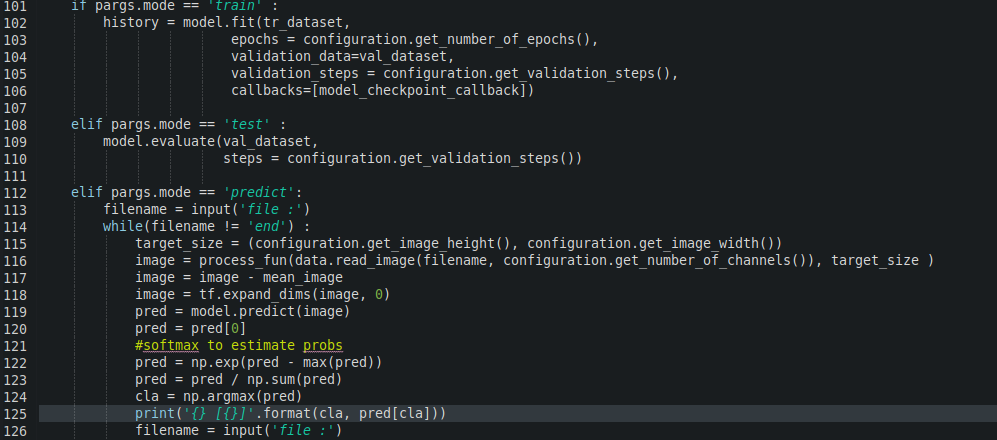EN
EN
ES